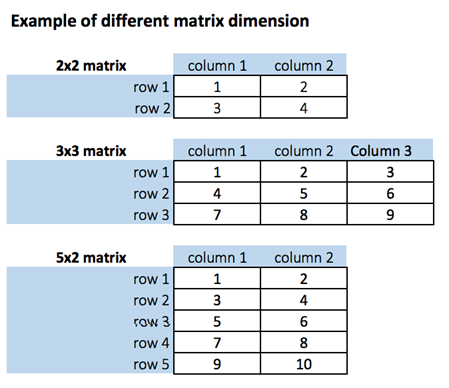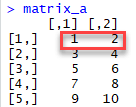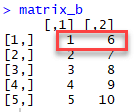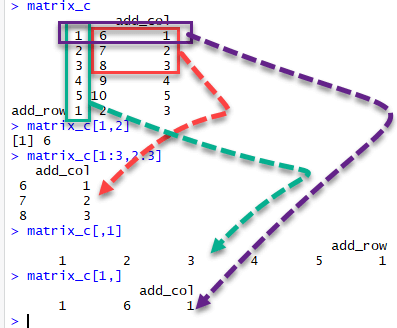# R tutorials for Business Analyst – R Matrix Create, Print, add Column, Slice

Hits: 90

## What is a Matrix?

A matrix is a 2-dimensional array that has m number of rows and n number of columns. In other words, matrix is a combination of two or more vectors with the same data type.

Note: It is possible to create more than two dimensions arrays with R.## How to Create a Matrix in R

We can create a matrix with the function matrix(). This function takes three arguments:

```matrix(data, nrow, ncol, byrow = FALSE)
```

Arguments:

• data: The collection of elements that R will arrange into the rows and columns of the matrix
• nrow: Number of rows
• ncol: Number of columns
• byrow: The rows are filled from the left to the right. We use `byrow = FALSE` (default values), if we want the matrix to be filled by the columns i.e. the values are filled top to bottom.

Let’s construct two 5×2 matrix with a sequence of number from 1 to 10, one with byrow = TRUE and one with byrow = FALSE to see the difference.

```matrix_a <-matrix(1:10, byrow = TRUE, nrow = 5)
matrix_a
```

Output:#### Print dimension of the matrix with dim()

```# Print dimension of the matrix with dim()
dim(matrix_a)```

Output:

`##  5 2`

#### Construct a matrix with 5 rows that contain the numbers 1 up to 10 and byrow = FALSE

```# Construct a matrix with 5 rows that contain the numbers 1 up to 10 and byrow =  FALSE
matrix_b <-matrix(1:10, byrow = FALSE, nrow = 5)
matrix_b```

Output:#### Print dimension of the matrix with dim()

```# Print dimension of the matrix with dim()
dim(matrix_b)```

Output:

`##  5 2`

Note: Using command matrix_b <-matrix(1:10, byrow = FALSE, ncol = 2) will have same effect as above.

You can also create a 4×3 matrix using ncol. R will create 3 columns and fill the row from top to bottom. Check an example

```matrix_c <-matrix(1:12, byrow = FALSE, ncol = 3)
matrix_c```

Output:

```##       [,1] [,2] [,3]
## [1,]    1    5    9
## [2,]    2    6   10
## [3,]    3    7   11
## [4,]    4    8   12
```

Example:

`dim(matrix_c)`

Output:

`##  4 3`

## Add a Column to a Matrix with the cbind()

You can add a column to a matrix with the cbind() command. cbind() means column binding. cbind()can concatenate as many matrix or columns as specified. For example, our previous example created a 5×2 matrix. We concatenate a third column and verify the dimension is 5×3

Example:

```# concatenate c(1:5) to the matrix_a
matrix_a1 <- cbind(matrix_a, c(1:5))
# Check the dimension
dim(matrix_a1)
```

Output:

`##  5 3`

Example:

`matrix_a1`

Output

```##       [,1] [,2] [,3]
## [1,]    1    2    1
## [2,]    3    4    2
## [3,]    5    6    3
## [4,]    7    8    4
## [5,]    9   10    5

```

Example:

We can also add more than one column. Let’s see the next sequence of number to the matrix_a2 matrix. The dimension of the new matrix will be 4×6 with number from 1 to 24.

`matrix_a2 <-matrix(13:24, byrow = FALSE, ncol = 3)`

Output:

```##      [,1] [,2] [,3]
## [1,]   13   17   21
## [2,]   14   18   22
## [3,]   15   19   23
## [4,]   16   20   24
```

Example:

```matrix_c <-matrix(1:12, byrow = FALSE, ncol = 3)
matrix_d <- cbind(matrix_a2, matrix_c)
dim(matrix_d)```

Output:

`##  4 6`

NOTE: The number of rows of matrices should be equal for cbind work

cbind()concatenate columns, rbind() appends rows. Let’s add one row to our matrix_c matrix and verify the dimension is 5×3

```matrix_c <-matrix(1:12, byrow = FALSE, ncol = 3)
# Create a vector of 3 columns
# Append to the matrix
# Check the dimension
dim(matrix_c)
```

Output:

`##  5 3`

## Slice a Matrix

We can select elements one or many elements from a matrix by using the square brackets [ ]. This is where slicing comes into the picture.

For example:

• matrix_c[1,2] selects the element at the first row and second column.
• matrix_c[1:3,2:3] results in a matrix with the data on the rows 1, 2, 3 and columns 2, 3,
• matrix_c[,1] selects all elements of the first column.
• matrix_c[1,] selects all elements of the first row.

Here is the output you get for the above codes# Personal Career & Learning Guide for Data Analyst, Data Engineer and Data Scientist

## Applied Machine Learning & Data Science Projects and Coding Recipes for Beginners

A list of FREE programming examples together with eTutorials & eBooks @ SETScholars

# Projects and Coding Recipes, eTutorials and eBooks: The best All-in-One resources for Data Analyst, Data Scientist, Machine Learning Engineer and Software Developer

Topics included: Classification, Clustering, Regression, Forecasting, Algorithms, Data Structures, Data Analytics & Data Science, Deep Learning, Machine Learning, Programming Languages and Software Tools & Packages.
(Discount is valid for limited time only)`Disclaimer: The information and code presented within this recipe/tutorial is only for educational and coaching purposes for beginners and developers. Anyone can practice and apply the recipe/tutorial presented here, but the reader is taking full responsibility for his/her actions. The author (content curator) of this recipe (code / program) has made every effort to ensure the accuracy of the information was correct at time of publication. The author (content curator) does not assume and hereby disclaims any liability to any party for any loss, damage, or disruption caused by errors or omissions, whether such errors or omissions result from accident, negligence, or any other cause. The information presented here could also be found in public knowledge domains.`

# Learn by Coding: v-Tutorials on Applied Machine Learning and Data Science for Beginners

Please do not waste your valuable time by watching videos, rather use end-to-end (Python and R) recipes from Professional Data Scientists to practice coding, and land the most demandable jobs in the fields of Predictive analytics & AI (Machine Learning and Data Science).

The objective is to guide the developers & analysts to “Learn how to Code” for Applied AI using end-to-end coding solutions, and unlock the world of opportunities!